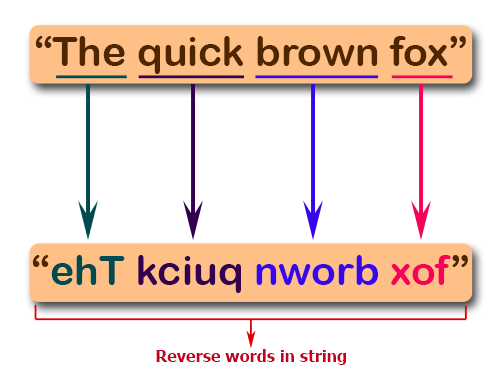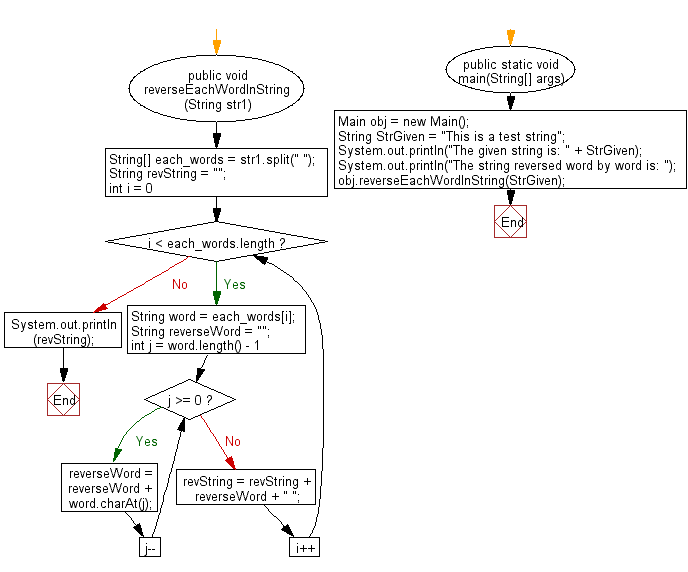﻿ Java exercises: Reverse every word in a string using methods - w3resource# Java String Exercises: Reverse every word in a string using methods

## Java String: Exercise-46 with Solution

Write a Java program to reverse every word in a string using methods.

Pictorial Presentation:Sample Solution:

Java Code:

``````import java.util.*;
public class Main {
public void reverseEachWordInString(String str1) {
String[] each_words = str1.split(" ");
String revString = "";
for (int i = 0; i < each_words.length; i++) {
String word = each_words[i];
String reverseWord = "";
for (int j = word.length() - 1; j >= 0; j--) {
reverseWord = reverseWord + word.charAt(j);
}
revString = revString + reverseWord + " ";
}
System.out.println(revString);
}
public static void main(String[] args) {
Main obj = new Main();
String StrGiven = "This is a test string";
System.out.println("The given string is: " + StrGiven);
System.out.println("The string reversed word by word is: ");
obj.reverseEachWordInString(StrGiven);
}
}
```
```

Sample Output:

```The given string is: This is a test string
The string reversed word by word is:
sihT si a tset gnirts
```

Flowchart:Java Code Editor:

Improve this sample solution and post your code through Disqus

What is the difficulty level of this exercise?

﻿

New Content: Composer: Dependency manager for PHP, R Programming